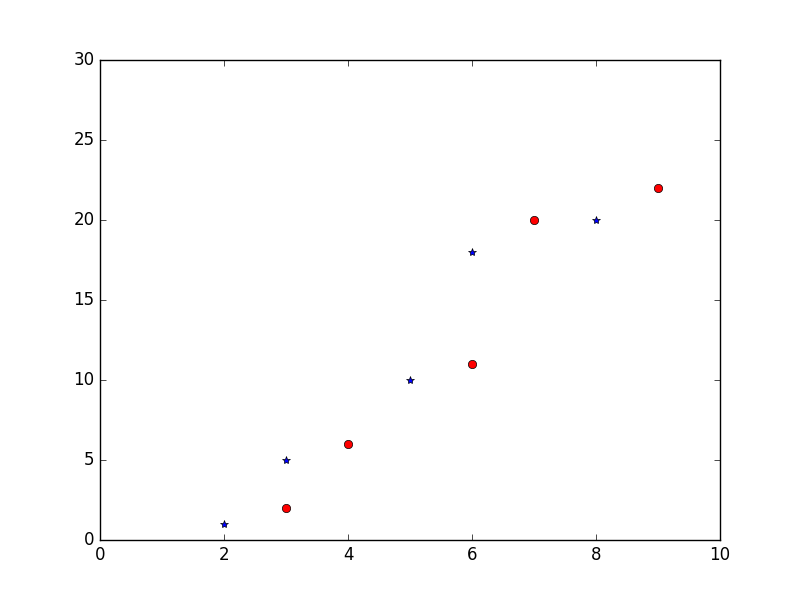﻿ Matplotlib Basic: Plot quantities which have an x and y position - w3resource# Matplotlib Basic: Plot quantities which have an x and y position

## Matplotlib Basic: Exercise-10 with Solution

Write a Python program to plot quantities which have an x and y position.

Sample Solution:

Python Code:

``````import numpy as np
import pylab as pl
# Make an array of x values
x1 = [2, 3, 5, 6, 8]
# Make an array of y values for each x value
y1 = [1, 5, 10, 18, 20]
# Make an array of x values
x2 = [3, 4, 6, 7, 9]
# Make an array of y values for each x value
y2 = [2, 6, 11, 20, 22]
# set new axes limits
pl.axis([0, 10, 0, 30])
# use pylab to plot x and y as red circles
pl.plot(x1, y1,'b*', x2, y2, 'ro')
# show the plot on the screen
pl.show()
```
```

Sample Output:Python Code Editor: